# Maharashtra Board Class 9 Maths Solutions Chapter 2 Real Numbers Problem Set 2

## Maharashtra State Board Class 9 Maths Solutions Chapter 2 Real Numbers Problem Set 2

Question 1.
Choose the correct alternative answer for the questions given below. [1 Mark each]

i. Which one of the following is an irrational number?√5

ii. Which of the following is an irrational number?
(A) 0.17
(B) $$1.\overline { 513 }$$
(C) $$0.27\overline { 46 }$$
(D) 0.101001000……..
(D) 0.101001000……..

iii. Decimal expansion of which of the following is non-terminating recurring?(C) $$\frac { 3 }{ 11 }$$

iv. Every point on the number line represents which of the following numbers?
(A) Natural numbers
(B) Irrational numbers
(C) Rational numbers
(D) Real numbers
(D) Real numbers

v. The number [/latex]0.\dot { 4 }[/latex] in $$\frac { p }{ q }$$ form is ……(A) $$\frac { 4 }{ 9 }$$

vi. What is √n , if n is not a perfect square number ?
(A) Natural number
(B) Rational number
(C) Irrational number
(D) Options A, B, C all are correct.
(C) Irrational number

vii. Which of the following is not a surd ?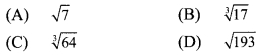(C) $$\sqrt [ 3 ]{ \sqrt { 64 } }$$

viii. What is the order of the surd $$\sqrt [ 3 ]{ \sqrt { 5 } }$$ ?
(A) 3
(B) 2
(C) 6
(D) 5
(C) 6

ix. Which one is the conjugate pair of 2√5 + √3 ?
(A) -2√5 + √3
(B) -2√5 – √3
(C) 2√3 – √5
(D) √3 + 2√5
(A) -2√5 + √3

x. The value of |12 – (13 + 7) x 4| is ____ .
(A) – 68
(B) 68
(C) – 32
(D) 32
(B) 68

Hints:
ii. Since the decimal expansion is neither terminating nor recurring, 0.101001000…. is an irrational number.

iii. $$\frac { 3 }{ 11 }$$
Denominator =11 = 1 x 11
Since, the denominator is other than prime factors 2 or 5.
∴ the decimal expansion of $$\frac { 3 }{ 11 }$$ will be non terminating recurring.

v. Let x = [/latex]0.\dot { 4 }[/latex]
∴10 x = [/latex]0.\dot { 4 }[/latex]
∴10 – x = [/latex]4.\dot { 4 }[/latex] – [/latex]0.\dot { 4 }[/latex]
∴9x = 4
∴ x = $$\frac { 4 }{ 9 }$$

vii. $$\sqrt{61}$$ = 4, which is not an irrational number.

viii. $$\sqrt{\sqrt{5}}=\sqrt[3 \times 2]{5}=\sqrt{5}$$
∴ Order = 6

ix. The conjugate of 2√5 + √3 is 2√5 – √3 or -2√5 + √3

x. |12 – (13+7) x 4| = |12 – 20 x 4|
= |12 – 80|
= |-68|
= 68

Question 2.
Write the following numbers in $$\frac { p }{ q }$$ form.
i. 0.555
ii. $$29.\overline { 568 }$$
iii. 9.315315…..
iv. 357.417417…..
v . $$30.\overline { 219 }$$
Solution:ii. Let x = $$29.\overline { 568 }$$ …(i)
x = 29.568568…
Since, three numbers i.e. 5, 6 and 8 are repeating after the decimal point.
Thus, multiplying both sides by 1000,
1000x = 29568.568568…
1000 x= $$29568.\overline { 568 }$$ …(ii)
Subtracting (i) from (ii),
1000x – x = $$29568.\overline { 568 }$$ – $$29.\overline { 568 }$$
∴ 999x = 29539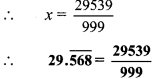iii. Let x = 9.315315 … = $$9.\overline { 315 }$$ …(i)
Since, three numbers i.e. 3, 1 and 5 are repeating after the decimal point.
Thus, multiplying both sides by 1000,
1000x = 9315.315315…
∴1000x = $$9315.\overline { 315 }$$ …(ii)
Subtracting (i) from (ii),
1000x – x = $$9315.\overline { 315 }$$ – $$9.\overline { 315 }$$
∴ 999x = 9306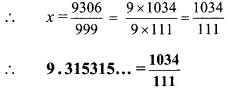iv. Let x = 357.417417… = $$357.\overline { 417 }$$ …(i)
Since, three numbers i.e. 4, 1 and 7 are repeating after the decimal point.
Thus, multiplying both sides by 1000,
1000x = 357417.417417…
∴ 1000x = 357417.417 …(ii)
Subtracting (i) from (ii),
1000x – x = $$357417.\overline { 417 }$$ – $$357.\overline { 417 }$$
∴ 999x = 357060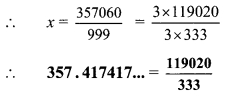v. Let x = $$30.\overline { 219 }$$ …(i)
∴ x = 30.219219
Since, three numbers i.e. 2, 1 and 9 are repeating after the decimal point.
Thus, multiplying both sides by 1000,
1000x= 30219.219219…
∴ 1000x = $$30219.\overline { 219 }$$ …(ii)
Subtracting (i) from (ii),
1000x – x = $$30219.\overline { 219 }$$ – $$30.\overline { 219 }$$
∴ 999x = 30189Question 3.
Write the following numbers in its decimal form.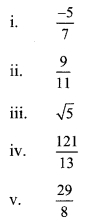Solution:
i. $$\frac { -5 }{ 7 }$$ii. $$\frac { 9 }{ 11 }$$iii. √5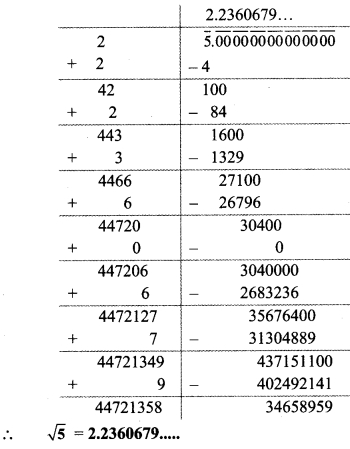iv. $$\frac { 121 }{ 13 }$$v. $$\frac { 29 }{ 8 }$$Question 4.
Show that 5 + √7 is an irrational number. [3 Marks]
Solution:
Let us assume that 5 + √7 is a rational number. So, we can find co-prime integers ‘a’ and ‘b’ (b ≠ 0) such thatSince, ‘a’ and ‘b’ are integers, $$\sqrt [ a ]{ b }$$ – 5 is a rational number and so √7 is a rational number.
∴ But this contradicts the fact that √7 is an irrational number.
Our assumption that 5 + √7 is a rational number is wrong.
∴ 5 + √7 is an irrational number.

Question 5.
Write the following surds in simplest form.Solution: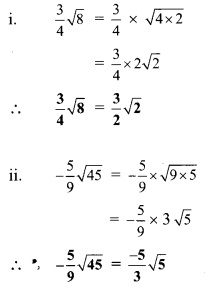Question 6.
Write the simplest form of rationalising factor for the given surds.Solution:Now, 4√2 x √2 = 4 x 2 = 8, which is a rational number.
∴ √2 is the simplest form of the rationalising factor of √32 .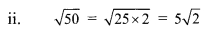Now, 5√2 x √2 = 5 x 2 = 10, which is a rational number.
∴ √2 is the simplest form of the rationalising factor of √50 .Now, 3√3 x √3 = 3 x 3 = 9, which is a rational number.
∴ √ 3 is the simplest form of the rationalising factor of √27 .= 6, which is a rational number.
∴ √10 is the simplest form of the rationalising factor of $$\sqrt [ 3 ]{ 5 }$$ √10 .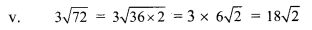Now, 18√2 x √2 = 18 x 2 = 36, which is a rational number.
∴ √2 is the simplest form of the rationalising factor of 3√72.

vi. 4√11
4√11 x √11 = 4 x 11 = 44, which is a rational number.
∴ √11 is the simplest form of the rationalising factor of 4√11.

Question 7.
Simplify.Solution: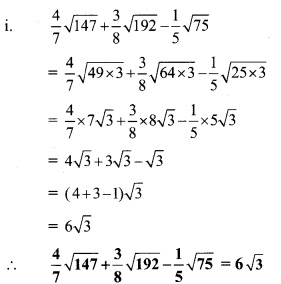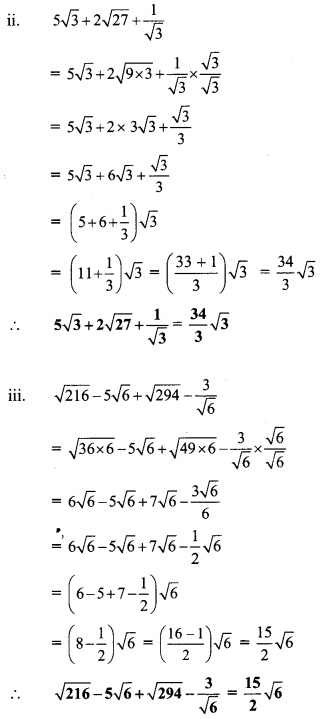Question 8.
Rationalize the denominator.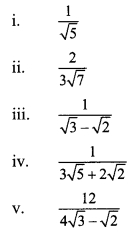Solution: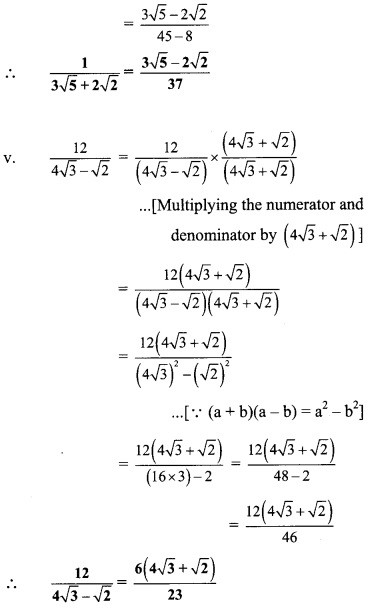Question 1.
Draw three or four circles of different radii on a card board. Cut these circles. Take a thread and measure the length of circumference and diameter of each of the circles. Note down the readings in the given table. (Textbook pg.no.23 )Solution:
i. 14,44,3.1
ii. 16,50.3,3.1
iii. 11,34.6,3.1
From table, we observe that the ratio $$\sqrt [ c ]{ d }$$ is nearly 3.1 which is constant. This ratio is denoted by π (pi).

Question 2.
To find the approximate value of π, take the wire of length 11 cm, 22 cm and 33 cm each. Make a circle from the wire. Measure the diameter and complete the following table.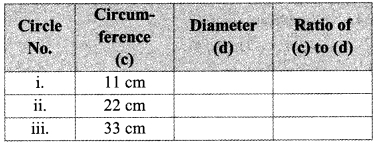Verify that the ratio of circumference to the diameter of a circle is approximately $$\sqrt [ 22 ]{ 7 }$$. (Textbook pg. no. 24)
Solution:
i. 3.5, $$\sqrt [ 22 ]{ 7 }$$
ii. 7, $$\sqrt [ 22 ]{ 7 }$$
iii. 10.5, $$\sqrt [ 22 ]{ 7 }$$
∴ The ratio of circumference to the diameter of each circle is $$\sqrt [ 22 ]{ 7 }$$.